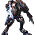#### 19 comments:

1.1.No man dey don't have no right angles.
"However they do not meet at right angles*

2.A)Parallelogram
B)Square
C)Triangle?(Not too sure for this. lol)
D)Trapezium

1.C is Rhombus, sorry. Didn't know there was only the 5 options.

2.C might be a kite too

3.This comment has been removed by the author.

1.This comment has been removed by the author.

4.This comment has been removed by the author.

1.This comment has been removed by the author.

5.a) Parallelogram
b) Square
c) Isosceles triangle
d) Trapezium

6.a) parallelogram
b)square
c)kite
d)trapezium

7.a) parallelogram
b) square
c) kite
d) trapezium

8.a) parallelogram
b) square
c) triangle
d)trapeizium

9.1) Rhombus

2) Rectangle

3) Kite

4 Trapezium

10.a) parallelogram
b) square
c) kite
d) trapezium

11.(a) Parallelogram
(b)Square
(c)kite
(d)trapezium

12.(a) Parallelogram
(b) Square
(c) Kite
(d) Trapezium

13.a) Parallelogram
b) Square
c) Kite
d) Trapezium

14.a)parallelogram
b)square
c) Unsure
d)Trapezium